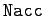Next: Validation of numerical solutions Up: The chaotic pendulum Previous: Analytic solution

## Numerical solution

In the following, we present numerical solutions of Eqs. (81) and (82) obtained using a fixed step-length, fourth-order, Runge-Kutta integration scheme. The step-length is conveniently parameterized by, which is defined as the number of time-steps taken by the integration scheme per period of the external drive.

Richard Fitzpatrick 2006-03-29